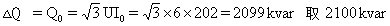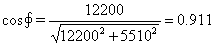# 大型风主变压器一电动机组使用并联电容器补偿无功案例

2020-09-21 15:26 库克库伯72tg=-1.02即Qf=-2000x（-1.02）=2040kvar

1.假定空载时全为无功消耗补偿值为2.按电机实际消耗的无功补偿即Q=Qf=2040kvar

3.按无功平衡进行补偿：

P1=14200kWQ1=6470kvar平均的cos1=0.91tg1=0.456

P2=14200-2000=12200kW

Q2=6470+2040=8510kvar

tg2=8510/12200=0.6975平均cos=0.82

△Q=12200x（0.6975-4556）=2951kvar取3000kvar